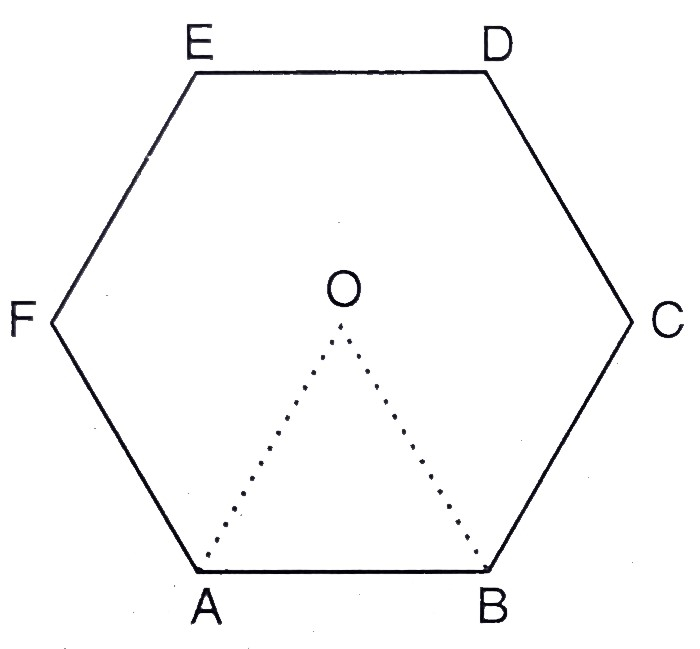"
">

# $A B C D E F$ is a regular hexagon with centre $O$. If the area of triangle $O A B$ is 9 $\mathrm{cm}^{2}$, find the area of the circle in which the hexagon is inscribed."

Given:

$A B C D E F$ is a regular hexagon with centre $O$.

The area of triangle $O A B$ is 9 $\mathrm{cm}^{2}$.

To do:

We have to find the area of the circle in which the hexagon is inscribed.

Solution:

We get six equal equilateral triangles by joining the vertices of the hexagon with $O$.

Area of the equilateral triangle $OAB = 9\ cm^2$.

This implies,

Area of hexagon $= 9 \times 6\ cm^2$

$= 54\ cm^2$

Let the radius of the circle $= OB =AB = r$

Therefore,

$\frac{\sqrt{3}}{4} r^{2}=9$

$\Rightarrow r^{2}=\frac{9 \times 4}{\sqrt{3}}$

$\Rightarrow r^{2}=\frac{36}{\sqrt{3}}$

Area of the circle $=\pi r^{2}$

$=\frac{22}{7} \times \frac{36}{\sqrt{3}}$

$=\frac{22 \times 36 \times \sqrt{3}}{7 \sqrt{3} \times \sqrt{3}}$

$=\frac{22 \times 36 \times \sqrt{3}}{7 \times 3}$

$=\frac{22 \times 12 \sqrt{3}}{7}$

$=\frac{264(1.73)}{7}$

$=\frac{456.72}{7}$

$=65.24 \mathrm{~cm}^{2}$

The area of the circle is $65.24 \mathrm{~cm}^{2}$.

Updated on: 10-Oct-2022

166 Views